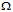# Digital Electronics - Digital Signal Processing

### Exercise :: Digital Signal Processing - Filling the Blanks

1.

An offset error in a DAC will show up as an incorrect analog output ________.

 A. only for higher value inputs B. only for lower value inputs C. only for certain (scattered) inputs D. for all inputs

Explanation:

No answer description available for this question. Let us discuss.

2.

An ADC that compares each bit, one at a time, with the input analog signal is a ________.

 A. single-slope ramp converter B. dual-slope ramp converter C. successive-approximation converter D. tracking converter

Explanation:

No answer description available for this question. Let us discuss.

3.

A standard logic device can be connected on a bus system as an open-collector logic device by connecting each output to a ________.

 A. discrete transistor B. 10 kseries resistor C. light-emitting diode D. CMOS buffer

Explanation:

No answer description available for this question. Let us discuss.

4.

A monotonicity error in a DAC will show up as an incorrect analog output ________.

 A. only for higher value inputs B. only for lower value inputs C. only for certain (scattered) inputs D. for all inputs

Explanation:

No answer description available for this question. Let us discuss.

5.

Of the methods listed, the fastest A/D conversion is done by a ________.

 A. single-slope ramp converter B. dual-slope ramp converter C. successive-approximation converter D. tracking converter